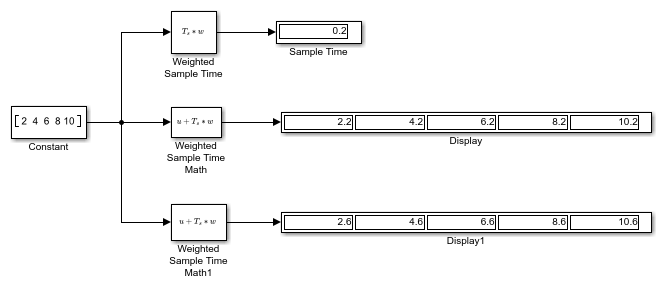Main Content

# Sample Time Math Operations Using the Weighted Sample Time Math Block

This example shows how to add the sample time value to a signal using the Weighted Sample Time Math block.Using the Weighted Sample Time block, you can see the sample time of this model is `0.2`. When you set the Operation parameter to `+` and the Weight value to `1` on the Weighted Sample Time Math block, the block adds the sample time value of `0.2` to the input signal. When you set the Weight value to `3` in the Weighted Sample Time Math1 block, the block adds `Ts*3` to the input signal, thus increasing each value by `0.6`.

## Support

#### Model-Based Design for Embedded Control Systems

Download white paper